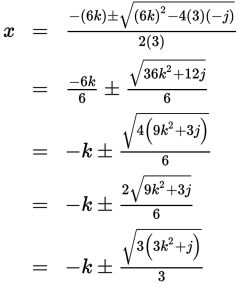# SAT Math Multiple Choice Question 937: Answer and Explanation

### Test Information

Question: 937

5. In the quadratic equation above, j and k are constants. What are the solutions for x?

• A.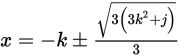• B.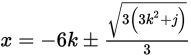• C.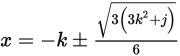• D.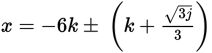Explanation:

A

Difficulty: Hard

Strategic Advice: When an equation involves fractions, it is usually best to clear the fractions first to avoid messy calculations later. Also, when the answer choices involve square roots, you'll need to use the quadratic formula,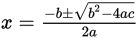.